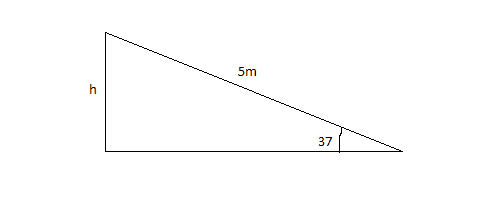# Change in Potential Energy

## Homework Statement

A 20 kg child slides down a slide at a playground. The slide is 5 meters long, and is inclined at an angle of 37 degrees above the horizontal. What is the child's change in potential?

## Homework Equations

PE=mgy

With m=mass, g=gravity, and y=distance.

## The Attempt at a Solution

I have solved potential energy problems before this,but I don't know how to account for the angle. If there was no angle, the formula would be PE=20*9.8*5, and I think that would be the change in energy since when the child reaches the bottom y=0, canceling out the entire equation. Could someone please tell me where the angle comes in? I don't want it done for me, I just need one post with a helpful explanation, and then I should be good. :)

Thanks so much!

Chestermiller
Mentor

## Homework Statement

A 20 kg child slides down a slide at a playground. The slide is 5 meters long, and is inclined at an angle of 37 degrees above the horizontal. What is the child's change in potential?

## Homework Equations

PE=mgy

With m=mass, g=gravity, and y=distance.

## The Attempt at a Solution

I have solved potential energy problems before this,but I don't know how to account for the angle. If there was no angle, the formula would be PE=20*9.8*5, and I think that would be the change in energy since when the child reaches the bottom y=0, canceling out the entire equation. Could someone please tell me where the angle comes in? I don't want it done for me, I just need one post with a helpful explanation, and then I should be good. :)

Thanks so much!
There are two ways of doing this that give the same answer.

Method 1: You use the equation you presented, but instead of the 5, you use the vertical distance that the child descended.

Method 2. You use the equation you presented, but instead of the 9.8, you use the tangential component of g along the slide.

Chet

•1 person
Thank you! I knew trig would come in somewhere, I just couldn't figure out how to bring it in. So using Method 1, would the vertical distance be 3.94 meters?

Chestermiller
Mentor
Thank you! I knew trig would come in somewhere, I just couldn't figure out how to bring it in. So using Method 1, would the vertical distance be 3.94 meters?
No. Try again. Draw a diagram this time.

Chet

•1 person
I drew one, but I did the trig wrong, I think. Would 5tan38 work?

Thanks again!

Chestermiller
Mentor
I drew one, but I did the trig wrong, I think. Would 5tan38 work?

Thanks again!
No. The hypotenuse is the slide. try again.

•1 person
Gold MemberFind h.
You won't need me here(Adjacent side)#### Attachments

•1 person
Hm, I'm not sure why I can't wrap my mind around this. It would have to be sine, but why? Wouldn't that be the two unknown sides?

Thanks!

Doc Al
Mentor
Hm, I'm not sure why I can't wrap my mind around this. It would have to be sine, but why? Wouldn't that be the two unknown sides?
What's the definition of sine for a right triangle?

Doc Al
Mentor
•1 person
Sine=opposite/hypotenuse

Oh! Obviously, 5 is the hypotenuse. I let adjacent's letter choice confuse me. So 5sin 37=3.0 m. Correct?

You may want to review some right triangle trig: Basic Trigonometric Functions

Thanks, I know the functions fairly well, but I tend to overcomplicate them. Oops.

Doc Al
Mentor
Sine=opposite/hypotenuse

Oh! Obviously, 5 is the hypotenuse. I let adjacent's letter choice confuse me. So 5sin 37=3.0 m. Correct?
Correct.

•1 person
PE=mgy
PE=20*9.8*3.0=588 Joules

Correct?

Thanks again!

Doc Al
Mentor
PE=mgy
PE=20*9.8*3.0=588 Joules

Correct?
Good. That's the magnitude of the change, but what's the sign of the change?

I forgot about this thread. Oops. The sign, as in negative or positive? It depends on what direction we decided is the positive direction, right? So if we decided that down was the positive direction, then h would be negative, so the answer would be negative? But can you have negative energy? Would I just say 588 Joules down?

Thanks!

Doc Al
Mentor
The sign, as in negative or positive?
Right.

It depends on what direction we decided is the positive direction, right? So if we decided that down was the positive direction, then h would be negative, so the answer would be negative? But can you have negative energy? Would I just say 588 Joules down?
It's got nothing to do with direction (energy is not a vector). It has to do with whether the potential energy increases (a positive change) or decreases (a negative change).

Since the child slides down the incline, is her change in PE positive or negative?

Her change in PE would be negative, so the KE would be positive.

Doc Al
Mentor
Her change in PE would be negative, so the KE would be positive.
Right. Since the change in PE is negative (the PE decreases), the change in KE will be positive (the KE increases). (The KE itself is always positive--unless it's zero.)

•1 person
Thank you!